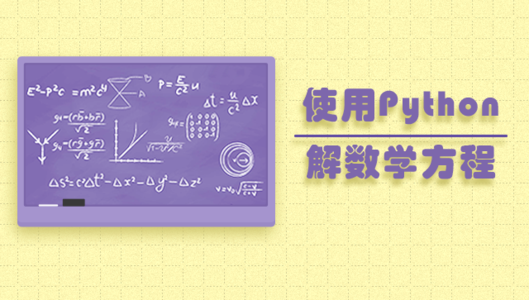• python基础知识

• 如何用SymPy库解线性方程组
• 如何用SymPy库解微积分相关习题（极限与积分）
• 如何用SymPy库解微分方程
• 如何用SymPy库化简矩阵

### 三、实验原理

SymPy是符号数学的Python库。它的目标是成为一个全功能的计算机代数系统，同时保持代码简洁、易于理解和扩展。 SymPy完全是用Python写的，并不需要外部的库。

``````#仅用于说明，不要直接运行
print x + y
``````

``````#仅用于说明，不要直接运行
x = Symbol('x')
y = Symbol('y')
print x + y
``````

### 四、实验步骤

#### SymPy库的安装

``````sudo pip install sympy
``````

#### 符号表示

``````from sympy import *
x = Symbol('x')
y = Symbol('y')
``````

``````from sympy import *
x, y = symbols('x y')
``````

``````x = Symbol('x1')
``````

#### 方程表示

• 加号 +
• 减号 -
• 除号 /
• 乘号 *
• 指数 **
• 对数 log()
• e的指数次幂 exp()

``````2 * x - y - 3 = 0
3 * x + y - 7 = 0
``````

#### 利用solve函数解方程

``````x * 2 - 4 = 0
``````

``````print solve(x * 2 - 4, x)
#result
#
``````

solve：第一个参数为要解的方程，要求右端等于0，第二个参数为要解的未知数。还有一些 其他的参数，想了解的可以去看官方文档。

``````solve([2 * x - y - 3, 3 * x + y - 7],[x, y])
``````

``````from sympy import *
x = Symbol('x')
y = Symbol('y')
print solve([2 * x - y - 3, 3 * x + y - 7],[x, y])
``````